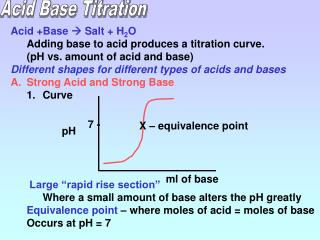DownloadDownload PresentationAcid Base Titration

# Acid Base Titration

Télécharger la présentation## Acid Base Titration

- - - - - - - - - - - - - - - - - - - - - - - - - - - E N D - - - - - - - - - - - - - - - - - - - - - - - - - - -
##### Presentation Transcript

1. Acid Base Titration • Acid +Base  Salt + H2O • Adding base to acid produces a titration curve. • (pH vs. amount of acid and base) • Different shapes for different types of acids and bases • Strong Acid and Strong Base • Curve • Large “rapid rise section” • Where a small amount of base alters the pH greatly • Equivalence point – where moles of acid = moles of base • Occurs at pH = 7 7 - X – equivalence point pH ml of base

2. 2. Determining pH of strong acid and base 1. Calculate the moles of acid or base used 2. Determine moles of acid or base left 3. convert leftover moles to Molarity 4. Use pH or pOH formula Example – If we add 500.0 ml of 0.1 M HBr to 225 ml of 0.2 M NaOH, what is the pH? 1. Calculate moles of H+ M = moles/L 0.1 = X 0.5 0.05 moles of H+ Subtract to get leftover moles of H+ 0.05 – 0.0450 = 0.0050 moles of H+ Convert to molarity 0.0050 0.500 + 0.225 2. Calculate moles of OH- M = moles/L 0.2 = X 0.225 0.0450 moles of OH- [H+] = 6.9 x 10-3M pH = 2.16

3. What is the pH of adding 50.0 ml of 2.0 M HCl to 55.0 ml of 1.0 M Ca(OH)2? 1. Calculate moles of H+ M = moles/L 2.0 = X 0.05 0.10 moles of H+ Subtract to get leftover moles of OH- 0.11 – 0.10 = 0.01 moles of OH- Convert to molarity 0.010 0.050 + 0.055 2. Calculate moles of OH- M = moles/L 1.0 = X 0.055 0.055 moles Moles of OH- = 2 x 0.055 Moles of OH- = 0.11 moles [OH-] = 0.0952 M pOH = 1.021 pH = 12.98